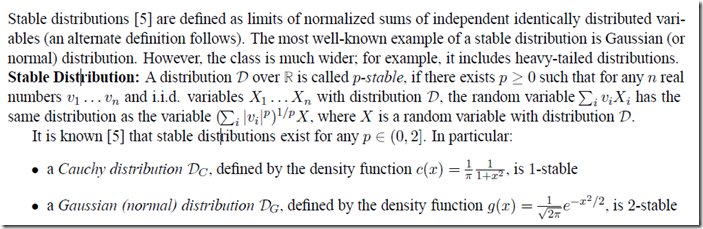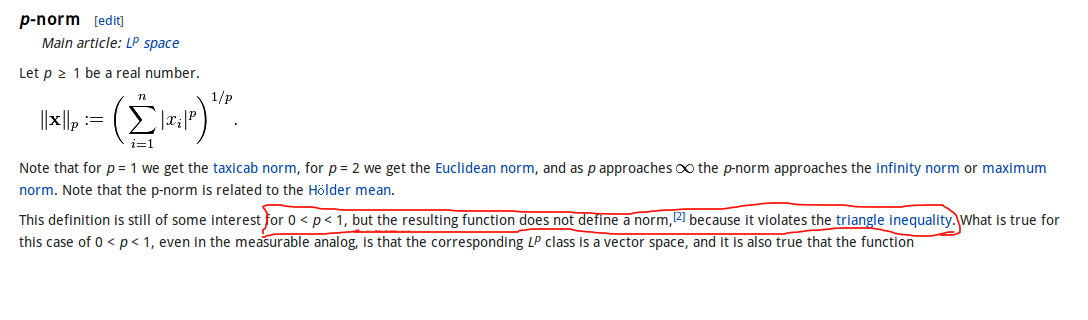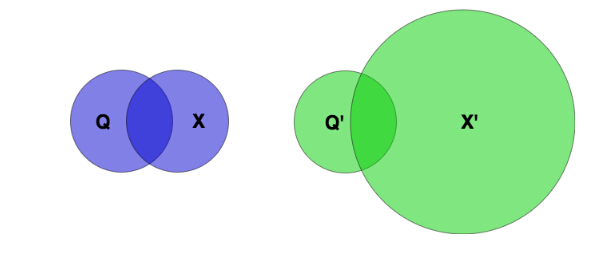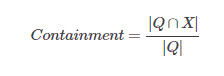• ## LSH

2011-04-01 16:11:00
Locality Sensitive Hashing collection code and sample data: http://people.csail.mit.edu/gregory/download.html<br /> Paper http://www.mit.edu/~andoni/LSH/

Locality Sensitive Hashing collection

code and sample data:

Paper
http://www.mit.edu/~andoni/LSH/
展开全文• LSH for Go 安装： go get github.com/ekzhu/lsh 该库包含用于L2度量空间中近似最近邻居搜索问题的各种本地敏感哈希（LSH）算法。 L2的LSH功能家族是的工作 目前包括：
• LSH源代码文件 LSH源代码文件 LSH源代码文件 LSH源代码文件
• LSH report for look up the performance of the algorithm
• 2015年7月4日，在LSHBOX-0.9中添加了一种新的LSH方法，即基于K均值的哈希双比特量化（KDBQ）。我们使用C ++实现KDBQ，但也提供了MATLAB接口。 Python界面将在以后添加到LSHBOX-0.9中。 与KDBQ相关的其他文件已同步...
• Lsh left shifts the integer part of the displayed value (multiplies it by 2) n times, where n is the next input number, and gives an integer result (base is set to Bin): 10 Lsh 3 = gives 80 (10 ...
Lsh left shifts the integer part of the displayed value (multiplies it by 2) n times, where n is the next input number, and gives an integer result (base is set to Bin):
10 Lsh 3 = gives 80 (10 multiplied by 2 three times).

10.345 Lsh 3 = also gives 80.


Rsh right shifts the value (performs an integer divide by 2) n times.
16 Rsh 2 = gives 4 (16 divided by 2 twice).

16.999 Rsh 2 = also gives 4.


Reference


展开全文C++
• p-stable LSH 背景 LSH 方法是处理海量高维数据 Approximate Nearest Neighbor（ANN）查询的有效的方法。 在处理欧氏空间中 ANN 问题时，原始的 LSH（Original LSH） 方法将原始空间中的点嵌入到 Hamming 空间中，...
文章目录p-stable LSH背景p-stable distributionp-stable LSH 的哈希函数两个向量在 p-stable LSH 的哈希函数映射下发生碰撞的概率分析E2LSH各路博客中 E2LSH 的解释一组哈希函数的情况多组哈希函数的情况实际上呢？需要 (r,c)-BC query 来回答 c-ANN query参考
p-stable LSH
背景
LSH 方法是处理海量高维数据 Approximate Nearest Neighbor（ANN）查询的有效的方法。
c-ANN 查询的定义为：给定一个查询点 $q$，且 $o^{*}$ 是精确的 NN 查询的结果，该查询返回一个点 $o$，$||o,q||_p \leq ||o^{*},q||_p$。
在处理欧氏空间中 ANN 问题时，原始的 LSH（Original LSH） 方法将原始空间中的点嵌入到 Hamming 空间中，即将欧氏空间中点的表示形式转换成 Hamming 空间中点的表示形式，原始空间中的距离度量转换成 Hamming 空间中的距离度量，即 Hamming距离（其定义为两个等长序列各位进行异或运算，结果为 1 的个数）。
对应该汉明距离的 Origin LSH 的为位采样运算（bit sampling）。但是一般距离都是用欧式距离进行度量的，将欧式距离映射到 Hamming 空间再比较其的 Hamming 距离比较麻烦，于是研究者提出了基于 p-stable distribution 的位置敏感哈希算法，不需要将原始空间嵌入到 Hamming 空间中而是可以直接处理欧式距离，并解决 (R,c)-近邻问题。
p-stable LSH应用在d维 lp-norm 下的欧几里得空间中，$p∈(0,2]$。
p-stable distribution
p-stable LSH 之所以会叫这个名字，是因为该算法应用到 p-stable distribution（p-稳定分布）的概念。下面给出的就是p-稳定分布的概念：
对于一个实数集 R 上的分布 D，如果存在 $p>=0$ ，对任何 n 个实数$v_1,…,v_n$和 n 个满足 D 分布的变量$X_1,…,X_n$，随机变量 $\sum_iv_iX_i$ 和 $(\sum_i|v_i|^p)^{1/p}X$ 有相同的分布，其中 $X$ 是服从D分布的一个随机变量，则称 D 为一个p稳定分布。
对任何 $p∈(0,2]$ 存在稳定分布：
$p=1$ 是柯西分布，概率密度函数为 $c(x)=\frac{1}{π(1+x^2)}$；
$p=2$ 是高斯分布，概率密度函数为 $g(x)=\frac{1}{(2π)^{\frac{1}{2}}}e^{-\frac{x^2}{2}}$。利用 p-stable distribution 可以有效的近似高维特征向量，并在保证度量距离的同时，对高维特征向量进行降维，这里先说是如何降维的，其关键思想是：

产生一个 d 维的随机向量 $a$，$a=(X_1,...,X_d)$，随机向量 $a$ 中的每一维随机的、独立的从 p-stable distribution中产生（每一个随机变量都满足 p-stable 分布）。
对于一个 d 维的特征向量 $v$，因为 $a \cdot v =\sum_iv_iX_i$，如 p-stable distribution 的定义，随机变量 $a \cdot v$ 具有和 $(\sum_i|v_i|^p)^{1/p}X$ 一样的分布，即和 $||v||_pX$ 是同分布的。可以用 $a \cdot v$ 表示向量 $v$ 来估算 $||v||_p$ ，其中 $||v||_p$ 表示向量 $v$ 在欧几里得空间 p-norm 下的长度（原始空间中的长度）。
上面 $a \cdot v$ 的结果是一个实数，就相当于将一个向量映射到了一个实数上，也就是一维上。我们可以用 k 个这样的随机向量 $\{a_i:a_i=(X_1,...,X_d), 1\leq i \leq k\}$，每一个随机向量都可以将这个向量映射到一维上，那么这 k 个随机向量映射得到的 k 个实数组成的向量，就可以看成是 k 维投影空间（降维了）中该向量的坐标。

这里证明 p=2 情形下，将高维数据映射到投影空间后，是如何保证距离度量的。p=2 采用欧氏距离作为距离度量方式，且记 p-stable distribution 服从标准正态分布，$X～N(0,1)$：

对于原始空间中任意两个特征向量 $v_1,v_2$，其在投影空间中的坐标点分别为 $v_1^{'},v_2^{'}$，记 $r=||v_1,v_2||$ 为其在原始空间中的距离，$r^{'}=||v_1^{'},v_2^{'}||$ 为其在投影空间中的距离。
记 $\rho_i$ 为投影空间中这两个点第 i 维坐标值的差，那么显然有 $r^{'} = (\sum_{i=1}^{k}\rho_i^{2})^{\frac{1}{2}}$。
由 p-stable 分布的定义可知，$\rho_i = a_i \cdot (v_1-v_2)$，与 $r \cdot X$ 是同分布的，即$\rho ～N(0,r^2)$，即$\frac{\rho}{r} ～ N(0,1)$。
$\frac{r^{'2}}{r^2} = \frac{\sum\rho_i^{2}}{r^2}$，也就是 k 个相互独立的标准正态分布之和，有：
$\frac{r^{'2}}{r^2} ～ \chi^2(k)$
接下来对 $r^{'}$ 的置信区间进行估计，建立起 $r$ 与 $r^{'}$ 之间的强依赖关系。给定原始空间中的两个点 $o_1,o_2$，我们有：

$The\ probability\ that\ r^{'}\ <\ r\sqrt{\chi_{1-\alpha}^{2}(k)}\ is\ \alpha.$
$The\ probability\ that\ r^{'}\ >\ r\sqrt{\chi_{\alpha}^{2}(k)}\ is\ \alpha.$
证明如下：对于卡方分布 $\chi^2(k)$ 来说，给定一个概率 $\alpha$，去寻找使得 $\chi^2(k)$ 大于某个数的概率为 $\alpha$，这个数就记为卡方分布在参数 $k,\alpha$ 下的上侧分位数，记为 $\chi^2_{\alpha}(k)$，表示：
$\int_{\chi^2_{\alpha}(k)}^{\infin}f(x;k) = \alpha$
我们知道 $\frac{r^{'2}}{r^2}$ 是服从卡方分布的，我们对 $\frac{r^{'2}}{r^2}$ 的置信区间 $I=[u,v]$ 进行估计，对任何给定的 $\alpha$ 要求其落在 $I$ 内的概率为 $1-2\alpha$。一个标准做法就是选择使得 $Pr[\frac{r^{'2}}{r^2}，即 $Pr[\frac{r^{'2}}{r^2}>u]=1-\alpha$ 以及 $Pr[\frac{r^{'2}}{r^2}>v]=\alpha$ 成立的 $u,v$取值，也就是找使得 $\int_{u}^{\infin}f(x;k) =1- \alpha$ 及 $\int_{v}^{\infin}f(x;k) = \alpha$ 成立的 $u,v$ 取值。根据卡方分布上侧分位数的定义，我们有 $u=\chi_{1-\alpha}^{2}(k)$ 和 $v=\chi_{\alpha}^{2}(k)$。所以:
$Pr[r^{'}r\sqrt{\chi_{\alpha}^{2}(k)}] = \alpha$
得证。这样就建立起了原始空间中的距离与投影距离的置信区间之间的一种强关系。

p-stable LSH 的哈希函数
在 p-stable LSH 中，点积 $a \cdot v$ 不用来估计 $||v||_p$ 的值，而是用来生成哈希函数，且该哈希函数是局部敏感的（即空间中距离较近的点映射后发生冲突的概率高，空间中距离较远的点映射后发生冲突的概率低），使用它对每一个特征向量 $v$ 赋予一个哈希值。
对于两个向量$v_1,v_2$$，(a \cdot v_1-a \cdot v_2)$是映射后的距离，而其值与 $||v_1-v_2||_pX$ 同分布，因此原始距离 $||v_1-v_2||_p$ 较小时，映射后的距离也小，因此使用点积来生成哈希函数可以保持局部敏感性。如果 $v_1$ 和 $v_2$ 距离很近，它们的哈希值将相同，并被哈希到同一个桶中的概率会很大。
大体方法：$a \cdot v$将特征向量 $v$ 映射到实数集R，如果将实轴以宽度 $w$ 等分，并对每一段进行标号，则 $a \cdot v$ 落到哪个区间，就将此区间标号作为哈希值赋给它。
哈希函数定义如下：
$h_{a,b}(v):R^d→ N$，映射一个 d 维特征向量 $v$ 到一个整数集。哈希函数中有两个随机变量 $a$ 和 $b$，其中 $a$ 为一个 d 维向量，随机向量 $a$ 中的每一维随机的、独立的从 p-stable distribution 中产生，$b$ 为 $[0,w]$ 范围内的随机数，$w$ 是人为设定的一个参数。对于一个固定的 $a,b$，p-stable LSH 的哈希函数 $h_{ab}(v)$ 为:
$h_{ab}(v) = \lfloor \frac{a \cdot v + b}{w} \rfloor$
这样的哈希函数构成的集合 $\{h_{a,b}(v):R^d→ N\}$ 为 p-stable LSH 的哈希函数族。
两个向量在 p-stable LSH 的哈希函数映射下发生碰撞的概率分析
从 p-stable LSH 的哈希函数族中随机选取一个哈希函数，现在估计两个向量 $v_1,v_2$ 在该哈希函数下发生冲突（也就是落在一个桶中）的概率。若要向量 $v_1$ 和 $v_2$ 映射后发生冲突，需要满足如下条件： $v_1$ 和 $v_2$ 通过与 $a$ 进行点积运算分别映射到一段长度为 $w$ 线段后，再通过加 $b$ 运算，能使映射后的点在同一条线段上。
首先定义 $c=||v_1-v_2||_p$（原始空间中的距离），$f_p(t)$为 p-stable 的分布的概率密度函数，那么特征向量 $v_1$ 和 $v_2$ 映射到一个桶上的距离是 $|a·v_1-a·v_2|，即$|(v_1-v_2)·a|，根据p-Stable分布的特性，即 $||v1-v2||_pX=|cX|，其中随机变量 $X$ 满足 p-stable distribution。
$P(c)=Pr[h_{ab}(v_1)-h_{ab}(v_2)] = \int_{0}^{w}\frac{1}{c}f_p(\frac{t}{c})(1-\frac{t}{w})dt$
关于这个概率公式的证明，详见它的最后一部分。
根据该式，可以得出两个特征向量的冲突碰撞概率随着距离 $c$（指的是原始空间中的距离） 的增加而减小，这符合局部敏感哈希函数的要求。
E2LSH
各路博客中 E2LSH 的解释
这里已经尽力把网上给出的 E2LSH 算法整理了一遍了，完全是没有道理的，至于为什么没有道理，在下一节开始会给出。
欧氏局部敏感哈希(E2LSH，Exact Euclidean locality sensitive Hashing)是 p-stable LSH在欧氏空间的一种随机化实现方法，其基本原理是：利用基于p-稳定分布的位置敏感函数对高维数据进行降维映射，使原始空间中距离很近的两个点经映射操作后依然很近。
一组哈希函数的情况
只有一个 $g(v)$ 的情况，只有一个哈希表。
为拉大距离近的点与距离远的点经映射后碰撞概率之间的差距，E2LSH 常将 k 个 p-stable LSH 哈希函数联合使用。
从 p-stable LSH 哈希函数族 $\{h_{a,b}(v):R^d→ N\}$ 选择 k 个哈希函数，组成一组哈希函数 $g(v)=(h_1(v),...,h_k(v))$，对于每个数据点 $v \in R^d$，经函数 $g(v)$ 降维后可以得到一个 k 维向量 $a = (a_1 , a_2 , ··· , a_k )$，这个 $k$ 元组就代表一个桶。
但将 k 元组 $a$ 直接作为该哈希表的桶标号，即占用内存，又不便于查找。为解决此问题，设计者又将其分层，使用数组+链表的方式，其中链表中的每一项都是一个哈希桶，即将原来的哈希表组织成下面的形式。对每个形式为 k 元组的桶标号 $a$，使用如下两个哈希函数 H1 和 H2 计算得到两个值：

其中 H1 的值是数组中的位置，数组的大小也就相当于是哈希表的大小。
其中 H2 的值作为 k 元组的代表，链接到对应 H1 数组位置的链表中。

H1 和H2 的具体形式如下：
$H_1(a) = [(\sum_{i=1}^{k}r_ia_i)\ mod\ C]\ mod\ tableSize \\ H_2(a) = (\sum_{i=1}^{k}r_i^{'}a_i)\ mod\ C$
其中:

$r_i$ 和 $r_i^{'}$ 是随机整数;
$C$ 是一个大素数，在 32 位机器上可以设置为 $2^{32}-5$;

索引构建过程：

对数据集中的所有点 $v$，使用 $g(v)$ 函数对其进行降维，也就是确定其桶标号。
然后使用新定义的哈希函数 $H_1$ 和 $H_2$，将其存在对应位置的哈希桶内
也就是根据 $H_1$ 的值找到在数组中的位置，然后根据 $H_2$ 的值在链表中寻找对应的哈希桶，在将原始数据点 $v$ 存到对应的哈希桶中。

查询过程如下：

对于查询点 q；
使用这 k 个哈希函数构成的函数（即 $g(q)$）计算桶标号的 k 元组；
对 k 元组计算 H1 和 H2 的值；
获取哈希表 H1 位置的链表；
在链表中查找 H2 值对应的哈希桶；
计算 q 与桶中样本的精确的距离，并排序；
找到规定的点。

多组哈希函数的情况
定义 $\{g_l(v):g_l(v)=(h_1(v),...,h_k(v)),1 \leq l \leq L\}$，也就是定义 $L$ 个前面那样的 $g(v)$ 函数，这对应着 $L$ 个哈希表。每个 $g_l(v)$ 函数由从哈希函数族 $\{h_{a,b}(v):R^d→ N\}$ 中随机独立地选取 k 个哈希函数组成，$g_l(v)$ 对向量 $v$ 降维后的值，表示其在第 $l$ 个哈希表中哈希桶标号。
对于每个 $g_l(v)$函数，都对应着一个哈希表，每个哈希表都由数组+链表的形式构成。在构建索引时，对数据集中的每个数据点 $v$，计算其 $L$ 个哈希函数的值，并将其存在 $L$ 个哈希表对应的哈希桶内。
查询时，同样计算查询点 $q$ 的 $L$ 个 $g_l(q)$ 函数值，找到 $q$ 所在的 $L$ 个哈希桶，计算 $q$ 与这些哈希桶中的全部点（有的说是 $3L$ 个点）的精确距离，找到规定的点。
实际上呢？需要 (r,c)-BC query 来回答 c-ANN query
实际上，现有的方法都不是都不是直接地去解决 c-ANN 查询问题的，因为就算你筛选出了候选集，但是你不知道精确的 NN 结果，所以你无法验证到底哪个点 $o$ 符合 $||q,o||\leq c*||q,o^{*}||$ 条件，所以上面的验证方法都是白扯。
为了解决 c-ANN 查询问题，提出了一个 $(r,c)-BC\ query（(r,c)\ ball\ cover）$ 的概念。这里给出它的定义，其中 $r$ 是超球体的半径，$c$ 是近似比，数据集为$D$，记 $B(q,r)$ 为以 $q$ 为中心，$r$ 为半径的一个超球体。一个 $(r,c)-BC$ 查询返回：

如果 $B(q,r)$ 至少包含 $D$ 中一个点的话，那么返回距离 $q$ 至多为 $cr$ 的一个点；
如果 $B(q,cr)$ 不包含 $D$ 中的任何点的话，那么什么都不返回。
$Otherwise,\ the\ result\ is\ undefined$.如上图，考虑 $(r,2)-BC$ 查询：

对 $q_1$ 查询时，由于 $B(q_1,r)$ 内有点，所以返回 $o_1$ 或 $o_2$，因为这两个点都在 $B(q_1,2r)$内；
对 $q_2$ 查询时，由于 $B(q_2,2r)$ 内没有点，所以什么都不返回。$c-ANN$ 查询可以通过执行一组 $(r,c)-BC$ 查询来解决。基本思想是这样的：如果$B(q,r)$ 不包含任何点，但 $B(q,cr)$ 包含点，那么 $B(q,cr)$ 中的任何一点都可以作为 $c-ANN$ 查询的结果。就是说精确解 $o^{*}$ 肯定是在这两个超球组成的超环之内的，所以对于这个超环内的任何一点 $o$ 肯定有 $||q,o||\leq ||q,o^{*}||$。如上图，$B(q,r)$ 不包含任何点，但是 $B(q,cr)$ 包含点，所以 $q$ 精确的 $NN$ 查询结果到 $q$ 的距离一定在 $r$ 和 $cr$ 之间，所以说 $B(q,cr)$ 中任何一点都是 $q$ 的 $c-ANN$ 查询的结果。
上一节中的方法进行 $(r,c)-BC query$ 是完全没问题的，但是直接回答 $c-ANN$ 查询是完全没有道理的，没有任何理论保证。
其实 LSH 的实际实现方法中，都是通过求解一系列的 $(r,c)-BC\ query$ 来解决 $c-ANN$ 问题的，除了E2LSH之外，还有PM_LSH，LSB-tree，C2LSH等。详细算法都需要涉及到复杂的理论保证推导，感兴趣自行了解。
参考
http://blog.sina.com.cn/s/blog_67914f2901019p3v.html。
https://blog.csdn.net/jasonding1354/article/details/38237353。
https://wenku.baidu.com/view/c616e7c008a1284ac85043cd.html。
https://www.cnblogs.com/jiejnan/archive/2012/03/13/2393660.html。
https://www.cnblogs.com/hxsyl/p/4627477.html。
https://blog.csdn.net/camu7s/article/details/48948069。


展开全文大数据
• LSH的开源代码，希望能对大家有点帮助。主要是局部哈希
• 关于局部敏感哈希算法，之前用R语言实现过，但是由于在R中效能太低，于是放弃用LSH来做相似性检索。学了Python发现很多模块都能实现，而且通过随机投影森林让查询数据更快，觉得可以试试大规模应用在数据相似性检索+...
关于局部敏感哈希算法，之前用R语言实现过，但是由于在R中效能太低，于是放弃用LSH来做相似性检索。学了Python发现很多模块都能实现，而且通过随机投影森林让查询数据更快，觉得可以试试大规模应用在数据相似性检索+去重的场景。

私认为，文本的相似性可以分为两类：一类是机械相似性；一类是语义相似性。
机械相似性代表着，两个文本内容上的相关程度，比如“你好吗”和“你好”的相似性，纯粹代表着内容上字符是否完全共现，应用场景在：文章去重；
语义相似性代表着，两个文本语义上的相似程度，比如“苹果”和“公司”的相似性，本篇不做这一讨论

之前写关于R语言实现的博客： R语言实现︱局部敏感哈希算法（LSH）解决文本机械相似性的问题（一，基本原理） R语言实现︱局部敏感哈希算法（LSH）解决文本机械相似性的问题（二，textreuse介绍）
.
机械相似性python版的四部曲： LSH︱python实现局部敏感随机投影森林——LSHForest/sklearn（一） LSH︱python实现局部敏感哈希——LSHash（二） 相似性︱python+opencv实现pHash算法+hamming距离（simhash）（三） LSH︱python实现MinHash-LSH及MinHash LSH Forest——datasketch（四）

HyperLogLog
HyperLogLog++
MinHash LSH
MinHash LSH Ensemble
MinHash LSH Forest
MinHash
Weighted MinHash
其中MinHash 与simHash不同，其主要采用的是Jaccard距离，LSHForest/sklearn是常规的Hash函数，所以可以用cosine距离。
其中，Jaccard距离较多地可以应用在BOW模型之上，图片和文字在用词袋模型表征特征的时候，较适合应用。
.

一、MinHash

在检索场景应用比较多，每当有新的搜索,需要创建一个新的MinHash，同时与候选集中求Jaccard相似性，然后根据一些阈值筛选符合的样例。

1、MinHash 主函数

class datasketch.MinHash(num_perm=128, seed=1, hashobj=<built-in function openssl_sha1>, hashvalues=None, permutations=None)

MinHash 哈希化专属的距离是 Jaccard距离。

num_perm (int, optional)
哈希置换函数设定个数，如果hashvalues有值，那么该参数将被忽略。

seed (int, optional)

— MinHash中随机种子。
hashobj (optional)

— MinHash的哈希方程式。
hashvalues (numpy.array or list, optional)

— 哈希内部状态。如果使用另外已经存在状态的MinHash，哈希初始化会更快
permutations (optional)

— 哈希置换函数的参数。如果有已经存在状态的MinHash，会更快
MinHash

2、MinHash案例

from datasketch import MinHash

data1 = ['minhash', 'is', 'a', 'probabilistic', 'data', 'structure', 'for',
'estimating', 'the', 'similarity', 'between', 'datasets']
data2 = ['minhash', 'is', 'a', 'probability', 'data', 'structure', 'for',
'estimating', 'the', 'similarity', 'between', 'documents']

m1, m2 = MinHash(), MinHash()
for d in data1:
m1.update(d.encode('utf8'))
for d in data2:
m2.update(d.encode('utf8'))
print("Estimated Jaccard for data1 and data2 is", m1.jaccard(m2))

s1 = set(data1)
s2 = set(data2)
actual_jaccard = float(len(s1.intersection(s2)))/float(len(s1.union(s2)))
print("Actual Jaccard for data1 and data2 is", actual_jaccard)

案例的大致步骤为：

MinHash初始化状态，需要预先设定MinHash()初始化
内容哈希化，内容m1.update哈希化
jaccard距离，float(len(s1.intersection(s2)))/float(len(s1.union(s2)))用集合的方式求距离
另外：

1、提高精度
m = MinHash(num_perm=256)

通过调整num_perm数量，来提高精度，代价是更多CPU消耗 + 更多内存

2、哈希合并
m1.merge(m2)

联合两个minhash，这样就可以更快的进行并行parallel MapReduce

3、求cardinality count
    def count(self):
k = len(self)
return np.float(k) / np.sum(self.hashvalues / np.float(_max_hash)) - 1.0

m.count()

二、MinHash LSH

LSH能够将相似的条例远大于非相似的，详细详细可见R语言实现︱局部敏感哈希算法（LSH）解决文本机械相似性的问题（一，基本原理）

1、主函数MinHash LSH

MinHashLSH(threshold=0.9, num_perm=128, weights=(0.5, 0.5), params=None)

threshold (float)

– Jaccard 距离阈值设定，默认为0.9
num_perm (int, optional)

– 哈希置换函数设定个数，在weighted-MinHash中为样本规模大小。
weights (tuple, optional)

– 优化Jaccard 阈值，能够弹性选择。
params (tuple, optional)

– bands 的数量与规模大小。
2、案例

from datasketch import MinHash, MinHashLSH

set1 = set(['minhash', 'is', 'a', 'probabilistic', 'data', 'structure', 'for',
'estimating', 'the', 'similarity', 'between', 'datasets'])
set2 = set(['minhash', 'is', 'a', 'probability', 'data', 'structure', 'for',
'estimating', 'the', 'similarity', 'between', 'documents'])
set3 = set(['minhash', 'is', 'probability', 'data', 'structure', 'for',
'estimating', 'the', 'similarity', 'between', 'documents'])

m1 = MinHash(num_perm=128)
m2 = MinHash(num_perm=128)
m3 = MinHash(num_perm=128)
for d in set1:
m1.update(d.encode('utf8'))
for d in set2:
m2.update(d.encode('utf8'))
for d in set3:
m3.update(d.encode('utf8'))

# Create LSH index
lsh = MinHashLSH(threshold=0.5, num_perm=128)
lsh.insert("m2", m2)
lsh.insert("m3", m3)
result = lsh.query(m1)
print("Approximate neighbours with Jaccard similarity > 0.5", result)

案例的大致步骤为：

MinHash初始化状态，需要预先设定MinHash(num_perm=128)初始化状态
内容哈希化，内容m1.update哈希化
MinHashLSH初始化， MinHashLSH(threshold=0.5, num_perm=128)
内容载入LSH系统，lsh.insert(“m3”, m3)，其中insert（Hash名称，minHash值）
查询，lsh.query，其中查询的内容也必须minHash化。
同时，MinHashLSH不能采用多项内容 Top-K 查询。可以使用之后的 MinHash LSH Forest，同时Jaccard 距离可能不是最好的选择，也可以选择其他的距离，可见MinHash LSH Ensemble.

额外的其他内容：

移除相关哈希值：
remove(key)

与lsh.insert(“m2”, m2)，对应。

是否为空
is_empty()

返回的是布尔值，检查hash是否为空
.

三、MinHash LSH Forest——局部敏感随机投影森林

与文章LSH︱python实现局部敏感随机投影森林——LSHForest/sklearn（一）类似，都是用来做随机投影森林的，这里专门使用minhash。

1、主函数

MinHashLSHForest(num_perm=128, l=8)

与原论文使用prefix trees不同，作者这里把哈希值存储在每个哈希列表中。
num_perm就是随机置换函数的个数，l代表prefix trees的数量。

其中每个前缀树的最大深度K取决于num_perm、l

k = int(num_perm / l)

.

2、案例

from datasketch import MinHashLSHForest, MinHash

data1 = ['minhash', 'is', 'a', 'probabilistic', 'data', 'structure', 'for',
'estimating', 'the', 'similarity', 'between', 'datasets']
data2 = ['minhash', 'is', 'a', 'probability', 'data', 'structure', 'for',
'estimating', 'the', 'similarity', 'between', 'documents']
data3 = ['minhash', 'is', 'probability', 'data', 'structure', 'for',
'estimating', 'the', 'similarity', 'between', 'documents']

# Create MinHash objects
m1 = MinHash(num_perm=128)
m2 = MinHash(num_perm=128)
m3 = MinHash(num_perm=128)
for d in data1:
m1.update(d.encode('utf8'))
for d in data2:
m2.update(d.encode('utf8'))
for d in data3:
m3.update(d.encode('utf8'))

# Create a MinHash LSH Forest with the same num_perm parameter
forest = MinHashLSHForest(num_perm=128)

# Add m2 and m3 into the index

# IMPORTANT: must call index() otherwise the keys won't be searchable
forest.index()

# Check for membership using the key
print("m2" in forest)
print("m3" in forest)

# Using m1 as the query, retrieve top 2 keys that have the higest Jaccard
result = forest.query(m1, 2)
print("Top 2 candidates", result)

案例的大致步骤为：

MinHash初始化状态，需要预先设定MinHash(num_perm=128)初始化状态
内容哈希化，内容m1.update哈希化
MinHashLSHForest初始化， MinHashLSHForest(num_perm=128)
forest.index()，相当于update一下，更新一下
查询，lsh.query，其中查询的内容也必须minHash化。
.
四、MinHash LSH Ensemble

新距离：Containment，简单介绍一下。案例一则：

from datasketch import MinHashLSHEnsemble, MinHash

set1 = set(["cat", "dog", "fish", "cow"])
set2 = set(["cat", "dog", "fish", "cow", "pig", "elephant", "lion", "tiger",
"wolf", "bird", "human"])
set3 = set(["cat", "dog", "car", "van", "train", "plane", "ship", "submarine",
"rocket", "bike", "scooter", "motorcyle", "SUV", "jet", "horse"])

# Create MinHash objects
m1 = MinHash(num_perm=128)
m2 = MinHash(num_perm=128)
m3 = MinHash(num_perm=128)
for d in set1:
m1.update(d.encode('utf8'))
for d in set2:
m2.update(d.encode('utf8'))
for d in set3:
m3.update(d.encode('utf8'))

# Create an LSH Ensemble index with a threshold
lshensemble = MinHashLSHEnsemble(threshold=0.8, num_perm=128)

# Index takes an iterable of (key, minhash, size)
lshensemble.index([("m2", m2, len(set2)), ("m3", m3, len(set3))])

# Check for membership using the key
print("m2" in lshensemble)
print("m3" in lshensemble)

# Using m1 as the query, get an result iterator
print("Sets with containment > 0.8:")
for key in lshensemble.query(m1, len(set1)):
print(key)

.

五、Weighted MinHash

Jaccard距离加权

import numpy as np

v1 = np.random.uniform(1, 10, 10)
v2 = np.random.uniform(1, 10, 10)
v3 = np.random.uniform(1, 10, 10)
mg = WeightedMinHashGenerator(10, 5)
m1 = mg.minhash(v1)
m2 = mg.minhash(v2)
m3 = mg.minhash(v3)

# Create weighted MinHash LSH index
lsh = MinHashLSH(threshold=0.1, sample_size=5)
lsh.insert("m2", m2)
lsh.insert("m3", m3)
result = lsh.query(m1)
print("Approximate neighbours with weighted Jaccard similarity > 0.1", result)
展开全文python minhash forest
• LSH Primer: https://github.com/FALCONN-LIB/FALCONN/wiki/LSH-Primer Github LSHash: https://github.com/kayzhu/LSHash 图像检索：再叙ANN Search:https://yongyuan.name/blog/ann-search.html Practical ...
• LSH算法 如果一篇文档由100维单词组成，LSH做的事情就是通过对维度分隔，从而对不同文档进行分桶。 如果我准备对100维度分成5个部分，每个部分对应20个维度。序列号为1，2，3，4，5 按照顺序来对比，如果1号中，两个...
• minhashLSH 1 问题场景 假设我们要找海量用户中哪些是行为相似的—— 用户A： id: 1001 name: 用户A data: “07:00 吃早餐，09:00 工作，12:00 吃午饭，13:00 打王者，18:00 吃晚饭，22:00 睡觉” mat: ...
• 负载均衡LSH 动机：经典的 LSH 构造了不平衡的结构，这自然会导致搜索效率低下。 虽然我们的负载平衡 LSH 获得了平衡的桶，所以 LBLSH 返回的图像候选的平均数量很少，从而大大加快了检测速度。 过程：初始化、...MATLAB
• LSH_内存 使用最近邻居搜索（NNS）和位置敏感哈希LSH的一键式学习 运行data_utils.py以下载和预处理Omniglot数据集 作者： 执行 其他参考
• 火花-LSH Spark 上的 LSH 实现。 这个想法基于 Coursera 上的斯坦福 MMD 课程。Scala
• ## E2LSH源代码

热门讨论 2014-08-01 17:02:24
E2LSH的源代码，p稳定分布LSH 用于大规模的图片检索
• Min-Hash-LSH-Python 在 Python 中重复数据删除的 Min Hash 和 LSH 的快速示例/实现Python
• 通过数据分析（包括局部敏感哈希（LSH））进行知识提取。 该存储库包含一个Jupyter笔记本，该笔记本使用数据集的子集测试4种不同类型的知识提取。 测试的知识提取方法为： 使用余弦相似度的“一对VS全部” 使用...
• LSH （Locality-sensitive-hashing）局部敏感哈希算法 matlab实现
• 最小哈希LSH MinHash 和 LSH 的 Java 实现，用于查找通过 Jaccard 相似度衡量的接近重复的文档。 MinHash 的实现，用于逼近文本文档中的 Jaccard 相似度。 还包括 LSH 的实现，这是一种快速查找近似最近邻的方法。Java
• 原始的LSH是基于hamming距离的，这里是基于欧式距离的LSH（E2LSH）C++代码欧式距离
• LSH Algorithm and Implementation (E2LSH) Locality-Sensitive Hashing (LSH) is an algorithm for solving the approximate or exact Near Neighbor Search in high dimensional spaces. This webpage ...局部敏感哈希 程序
• LSH邻居搜索技术介绍经典文档，详细的说明了NN搜搜的各种经典算法。
• LSH算法，简单介绍，发展历程，改进，所涉及的论文和参考资料。...